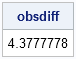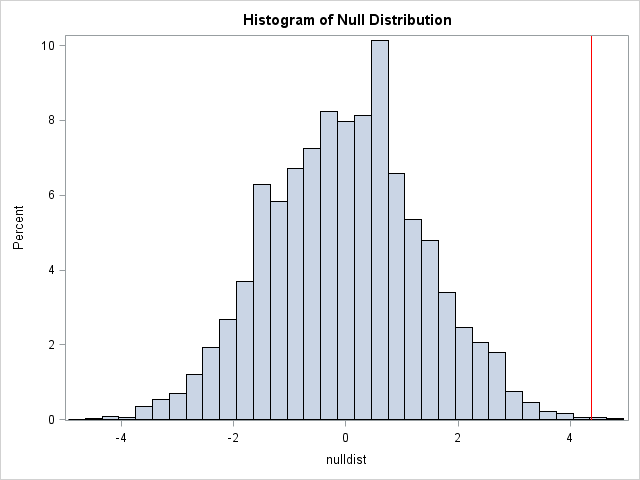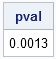My colleagues at the SAS & R blog recently posted an example of how to program a permutation test in SAS and R. Their SAS implementation used Base SAS and was "relatively cumbersome" (their words) when compared with the R code. In today's post I implement the permutation test in SAS/IML. This provides an apples-to-apples comparison because both SAS/IML and R are matrix-vector languages.

This permutation test is a simple resampling exercise that could be assigned as a homework problem in a classroom. If you are at a college or university, remember that SAS/IML is available for free for all academic users through the SAS University Edition.

### Permutation tests in SAS/IML

The analysis was motivated by a talk about using computational methods to illuminate statistical analyses. The data are the number of mosquitoes that were attracted to human volunteers in an experiment after each volunteer had consumed either a liter of beer (n=25) or water (n=18). The following statements assign the experimental data to two SAS/IML vectors and compute the observed difference between the means of the two groups:

```proc iml; G1 = {27, 19, 20, 20, 23, 17, 21, 24, 31, 26, 28, 20, 27, 19, 25, 31, 24, 28, 24, 29, 21, 21, 18, 27, 20}; G2 = {21, 19, 13, 22, 15, 22, 15, 22, 20, 12, 24, 24, 21, 19, 18, 16, 23, 20}; obsdiff = mean(G1) - mean(G2); print obsdiff;```The experimenters observed that, on average, people who drank beer attracted 4.4 more mosquitoes than people who drank water. The statistical question is, "What is the probability of observing a difference of this magnitude (or bigger) by chance if the beverages have no effect?" You can answer this question by using a permutation test to perform a nonparametric version of the t test. The null hypothesis is that there is no difference between the mean number of mosquitoes that were attracted to each experimental group (beer or water).

The permutation test enables you to generate the null distribution. Draw 25 random observations from the data and assign them to Group 1; assign the other 18 observations to Group 2. Compute the difference between the means of each group. Repeat these two steps many times to approximate the null distribution. The following SAS/IML statements use the SAMPLE function in SAS/IML to permute the data. The permutation step is repeated 9,999 times so that (adding in the original data order) there are a total of 10,000 permutations of the data:

```call randseed(12345); /* set random number seed */ alldata = G1 // G2; /* stack data in a single vector */ N1 = nrow(G1); N = N1 + nrow(G2); NRepl = 9999; /* number of permutations */ nulldist = j(NRepl,1); /* allocate vector to hold results */ do k = 1 to NRepl; x = sample(alldata, N, "WOR"); /* permute the data */ nulldist[k] = mean(x[1:N1]) - mean(x[(N1+1):N]); /* difference of means */ end;   title "Histogram of Null Distribution"; refline = "refline " + char(obsdiff) + " / axis=x lineattrs=(color=red);"; call Histogram(nulldist) other=refline;```The histogram shows the distribution of mean differences that were computed under the assumption of the null hypothesis. The observed difference between the beer and water groups (the vertical red line at 4.38) is way off in the tail. Since the null hypothesis is not a likely explanation for the observed difference, we reject it. We conclude that mosquitoes are attracted differently to the two groups (beer and water).

If you would like to compute the empirical p-value for the null distribution, that is easily accomplished:

```pval = (1 + sum(abs(nulldist) >= abs(obsdiff))) / (NRepl+1); print pval;```### Vectorization for permutation tests

Regular readers of my blog know that I advocate vectorizing programs whenever possible. Matrix-vector languages such as SAS/IML, R, and MATLAB work more efficiently when computations inside loops are replaced by vector or matrix computations.

Because of the way that SAS/IML loops are compiled and optimized, using loops in the SAS/IML language is not as detrimental to performance as in some other languages. For example, the previous permutation test code runs in about 0.04 seconds on my PC from 2009. Still, I like to promote vectorization because it can be important to performance.

The following statements eliminate the DO loop and implement the resampling and permutation test in two lines of SAS/IML code. The vectorized computation runs in about one-fourth the time:

```x = sample(alldata, N//NRepl, "WOR"); /* create all resamples */ nulldist = x[,1:N1][,:] - x[,(N1+1):N][,:]; /* compute all mean differences */```

The vectorized computation uses the colon (:) subscript reduction operator in SAS/IML to compute the mean of the first 25 and the last 18 elements for each set of permuted data.

### Additional references for resampling in SAS

To learn more about efficient ways to implement resampling methods such as bootstrapping and permutation tests, consult the following references:

• For information about bootstrapping in SAS/IML, see pages 14–17 of Wicklin (2008).
• For another permutation test example, see pages 11–14 of Wicklin (2012).
• Chapter 15 of my book Simulating Data with SAS describes resampling methods in both Base SAS and SAS/IML. I include useful tips and techniques that make bootstrapping in Base SAS less cumbersome, including a mention of the %BOOT and %BOOTCI macros, which enable you to implement bootstrap methods in Base SAS by using only a few program statements.
• For an excellent introduction to resampling methods in Base SAS, see Cassell (2007).
ShareDistinguished Researcher in Computational Statistics

Rick Wicklin, PhD, is a distinguished researcher in computational statistics at SAS and is a principal developer of SAS/IML software. His areas of expertise include computational statistics, simulation, statistical graphics, and modern methods in statistical data analysis. Rick is author of the books Statistical Programming with SAS/IML Software and Simulating Data with SAS.

1.Do you think any simulation by resampling could help me determine standard errors around cut scores for a math test?

Here is the background. Student performance is assigned to 1 of six categories of competence after a math achievement test using 5 cut scores. (If you think this is OK, I will send the table of 5 x 10 cut scores by email; the math score ranges from -2.88 to 2.77). A committee of six experts looked at the each of the 10 cut score sets and came up with the best pick he or she thought the best from the 10 cut score sets. Four tables summarizing performance data associated with the 10 cut score sets were the basis for the committee experts to review and serve as basis of their QUALITATIVE decision making. How can I simulate the way the committee worked to determine standard errors around the cut scores?

The committee of 6 experts made their evaluation, and 3 experts chose a specific cut score set ID=9 as the best cut score set, 1 expert chose cut score set ID=2 as the best cut score set, and 2 experts chose cut score set ID=3 as the best cut score set. Can I simulate this process n times via SAS to determine the standard error for each cut score?

•You can ask statistical questions and get help from SAS experts by posting to the SAS Statisitcal Procedures Support Community.

•Sure. Thanks, Rick.

2.I think it may be a little quicker to compute the mean differences as a matrix multiplication rather than using the mean subscript operator twice. It involves a lot more computation, but the advantage is not having to split the large matrix up.

y = j(N1, 1, 1/N1) // j(N-N1, 1, -1/(N-N1));
nulldist = sample(alldata, N//NRepl, "WOR") * y;

Does casting the problem as a matrix multiplication also have potential benefits from the multi-threading?

•Clever. Yes, there is a potential benefit to using matrix multiplication, which is multi-threaded.

3.Hey Rick,

I believe the original permutation test is to generate the sampling distribution under the null hypothesis by taking all possible combinations of the samples. This data might be a bit large to do, but how would you do it in theory? Something like (I know this won't work, but just thinking out loud):

*** Concatenate the data ***;
alldat = G1 // G2;

*** Generate the labels ***;
labels = repeat(0, nrow(G1)) // repeat(1, nrow(G2));

*** Create a matrix of permuted labels. ***;
perm_labels = allperm(labels)

*** Uh oh, allperm isnt correct. It generates duplicates since the permutations is n!. ***;
*** Need something like allcomb - but that isnt really what we want either. Need to get rid of those dups ***;

*** Create a loop or something to get the mean difference. Should be a good way to vectorize this. ***;

do i = 1 to ncol(perm_labels);
mm = mean(alldat[loc(perm_labels[:,i] = 0)]) - mean(alldat[loc(perm_labels[:,i] = 1)])
end;

I'm sure you have a better working solution than this.

•You are asking for an exact permutation test. Do an internet search for the keywords "exact permutation test in sas iml"

Be careful to distinguish the permutations of the elements from the combinations of the indices. A permutation test takes all PERMUTATIONS of the samples, so there will certainly be duplicates among the labels/indices. For example, if G1={1,2,3} and G2={4,5}, then a valid permutation is G1={3,2,1} and G2={5,4}. There are 5!=120 different permutations, even though there are only "5 choose 2"=10 unique pairs of indices. You have to use the number of permutations to get the p-values to come out correctly. If you write a program that takes distinct combinations of indices, be sure to adjust the counts by the appropriate multiplicity (12, in this example).

4.Efthymios Tsakonas on

Your blog is a source of inspiration to those who deal with computational statistics. Thank you. /Efthymios

5.Just comment on your friend on the blog, the datastep program may be simpler if using array,

```data bites;; if _n_ le 18 then drink="water"; else drink="beer"; infile datalines delimiter=','; input bites @@; datalines; 21, 19, 13, 22, 15, 22, 15, 22, 20, 12, 24, 24, 21, 19, 18, 16, 23, 20 27, 19, 20, 20, 23, 17, 21, 24, 31, 26, 28, 20, 27, 19, 25, 31, 24 28, 24, 29, 21, 21, 18, 27, 20 ;   proc sql noprint; select bites into : data separated by "," from bites; select count(bites) into : numobs from bites; select count(bites) into : water from bites where drink="water"; select count (bites) into : beer from bites where drink="beer"; run;   data temp; array x[&numobs] _TEMPORARY_ (&data); array y1[&water] _temporary_; array y2[&beer] _temporary_; seed=3987674;   do j=1 to dim(y1); y1[j]=x[j]; end;   do j=dim(y1)+1 to dim(x); y2[j-dim(y1)]=x[j]; end;   mainmean=mean(of y2[*])-mean(of y1[*]); call symput('mainmean', mainmean); do i=1 to 100000; call ranperm(seed, of x[*]); do j=1 to dim(y1); y1[j]=x[j]; end; do j=dim(y1)+1 to dim(y2); y2[j-dim(y1)]=x[j]; end; result=mean(of y2[*])-mean(of y1[*]); output; end; run;   proc sgplot data=temp; histogram result ; refline &mainmean/axis=x; run;```